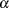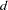The NLMIXED Procedure
 ESTIMATE Statement

ESTIMATE ’label’ expression <options> ;

The ESTIMATE statement enables you to compute an additional estimate that is a function of the parameter values. You must provide a quoted string to identify the estimate and then a valid SAS expression. Multiple ESTIMATE statements are permitted, and results from all statements are listed in a common table. PROC NLMIXED computes approximate standard errors for the estimates using the delta method (Billingsley 1986). It uses these standard errors to compute correspondingstatistics, p-values, and confidence limits.

The ECOV option in the PROC NLMIXED statement produces a table containing the approximate covariance matrix of all the additional estimates you specify. The ECORR option produces the corresponding correlation matrix. The EDER option produces a table of the derivatives of the additional estimates with respect to each of the model parameters.

The following options are available in the ESTIMATE statement:

ALPHA=specifies the alpha level to be used in computing confidence limits. The default value corresponds to the ALPHA= option in the PROC NLMIXED statement.

DF=specifies the degrees of freedom to be used in computing p-values and confidence limits. The default value corresponds to the DF= option in the PROC NLMIXED statement.Previous Page | Next Page | Top of Page